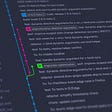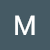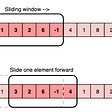# [Leet Code] Binary Gap

`Input: n = 22Output: 2Explanation: 22 in binary is "10110".The first adjacent pair of 1's is "10110" with a distance of 2.The second adjacent pair of 1's is "10110" with a distance of 1.The answer is the largest of these two distances, which is 2.Note that "10110" is not a valid pair since there is a 1 separating the two 1's underlined.`
`Input: n = 5Output: 2Explanation: 5 in binary is "101".`
`Input: n = 6Output: 1Explanation: 6 in binary is "110".`
`Input: n = 8Output: 0Explanation: 8 in binary is "1000".There aren't any adjacent pairs of 1's in the binary representation of 8, so we return 0.`
`Input: n = 1Output: 0`
`class Solution(object):    def binaryGap(self, n):        """        :type n: int        :rtype: int        """        binary = format(n, "b")        highest_num = 0        num_counter = 0        while num_counter < len(binary):            if binary[num_counter] == "1":                counter = 0                for i in range(num_counter+1, len(binary)):                    counter += 1                    if binary[i] == "1":                        if counter > highest_num:                             highest_num = counter                        num_counter = i-1                         break            num_counter += 1                                return highest_num`

--

--

--

Learning, and posting my findings!

Love podcasts or audiobooks? Learn on the go with our new app.

## Go-Kafka-Go## What do you need to know to work on industry-based projects(Android App Development)?## Web and Mobile Backend Services Patterns## Syncier Security Tower## Some unsolicited Old Man Advice## Getting Started with Git and Github## Matthew Boyd

Learning, and posting my findings!

## DS practice## Lowest Common Ancestor of a Binary Search Tree 🐳## [Leet Code] Maximum Number of Words Found in Sentences